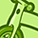# QlikView App Dev

Discussion Board for collaboration related to QlikView App Development.

Announcements
LINKEDIN LIVE: Democratizing data to enhance customer-centricity. JULY 29TH REGISTER TODAY
cancel
Showing results for
Did you mean:Not applicable

## Text objects with text linked on selections

I wonder if any one could help me.

I am trying to specify which currency the dashboard is in, depending on selections made. My effort of the script below -

='All figures in' &

if([_Currency]='Base' & [Entity ID]='1','GBP',0) or
if([_Currency]='Base' & [Entity ID]='2','SGD',0) or
if([_Currency]='Base' & [Entity ID]='3','AUD',0) or
if([_Currency]='Base' & [Entity ID]='4','HKD',0) or
if([_Currency]='Base' & [Entity ID]='5','USD',0) or
if([_Currency]='Base' & [Entity ID]='6','USD',0) or
if([_Currency]='Base' & [Entity ID]='7','CPLm',0) or
if([_Currency]='Base' & [Entity ID]='9','EUR',0) or
if([_Currency]='Base' & [Entity ID]='10','MEXP',0) or
if([_Currency]='Base' & [Entity ID]='11','BRL',0) or
if([_Currency]='Base' & [Entity ID]='12','USD',0) or
if([_Currency]='Base' & [Entity ID]='13','USD',0) or
if([_Currency]='Base' & [Entity ID]='14','COPm',0) or
if([_Currency]='Base' & [Entity ID]='15','EUR',0) or
if([_Currency]='Base' & [Entity ID]='16','EUR',0)

Many thanks.

John

1 Solution

Accepted SolutionsNot applicable
Author

i prefer something more dynamic but if you want to do it by if statement....

='All figures in'&

if([_Currency]='Base' and  [Entity_ID]='1','GBP',

if([_Currency]='Base' and [Entity_ID]='2','SGD',

if([_Currency]='Base' and [Entity_ID]='3','AUD',

if([_Currency]='Base' and [Entity_ID]='4','HKD',

if([_Currency]='Base' and [Entity_ID]='5','USD',

if([_Currency]='Base' and [Entity_ID]='6','USD',

if([_Currency]='Base' and [Entity_ID]='7','CPLm',

if([_Currency]='Base' and [Entity_ID]='9','EUR',

if([_Currency]='Base' and [Entity_ID]='10','MEXP',

if([_Currency]='Base' and [Entity_ID]='11','BRL',

if([_Currency]='Base' and [Entity_ID]='12','USD',

if([_Currency]='Base' and [Entity_ID]='13','USD',

if([_Currency]='Base' and [Entity_ID]='14','COPm',

if([_Currency]='Base' and [Entity_ID]='15','EUR',

if([_Currency]='Base' and [Entity_ID]='16','EUR',))))))))))))))))

2 RepliesNot applicable
Author

i prefer something more dynamic but if you want to do it by if statement....

='All figures in'&

if([_Currency]='Base' and  [Entity_ID]='1','GBP',

if([_Currency]='Base' and [Entity_ID]='2','SGD',

if([_Currency]='Base' and [Entity_ID]='3','AUD',

if([_Currency]='Base' and [Entity_ID]='4','HKD',

if([_Currency]='Base' and [Entity_ID]='5','USD',

if([_Currency]='Base' and [Entity_ID]='6','USD',

if([_Currency]='Base' and [Entity_ID]='7','CPLm',

if([_Currency]='Base' and [Entity_ID]='9','EUR',

if([_Currency]='Base' and [Entity_ID]='10','MEXP',

if([_Currency]='Base' and [Entity_ID]='11','BRL',

if([_Currency]='Base' and [Entity_ID]='12','USD',

if([_Currency]='Base' and [Entity_ID]='13','USD',

if([_Currency]='Base' and [Entity_ID]='14','COPm',

if([_Currency]='Base' and [Entity_ID]='15','EUR',

if([_Currency]='Base' and [Entity_ID]='16','EUR',))))))))))))))))Creator III

Hi,

You may use getfieldselections([Filed name]) function to get the currently selected value of a field.

Regards,

SomCommunity Browser